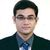QlikView App Dev

Discussion Board for collaboration related to QlikView App Development.

Announcements
QLIKWORLD LIVE! MAY 16 - 19TH, EARLY BIRD DISCOUNTS! REGISTER TODAY
cancel
Showing results for
Did you mean:Contributor III

inventory of a product based on the orders

Hi All,

I want to calculate the Inventory of the products based on its orders.

eg. in the source file, product P1 has total units has 30, its first order (Order id 1) has ordered 15 units so the remaining units of Prod P1 has 15, we call it as the order is completed. (30-15=15)

in the second-order  (Order id 2) it ordered 20 units, but we have 15 units only (15-20 =-5) partially completed.

in the third-order (Order id 3) it ordered 15 units, but we don't have units to give hence we canceled the order.

Sra1

Labels (2)

• New To Qlik

2 Solutions

Accepted SolutionsMVP

Hi,

Try this code.

Data:
ordid,
[noof orders],
[total units],
AutoNumber(RowNo(),prodid) as Rownum
FROM
(ooxml, embedded labels, table is Sheet1);

Left join (Data)
Load prodid, Sum([total units]) as [Overall Units]
Resident Data Group by prodid;

Data1:
Load *,If([Remaining Units]>0,'Completed',If([Remaining Units]<0,'Partial Completed','Cancelled')) as Status;
Load *,If(Rownum=1,[Overall Units]-[noof orders],If(peek([Remaining Units]) <=0,0,peek([Remaining Units])-[noof orders])) as [Remaining Units]
Resident Data Order by prodid,ordid;

Drop table Data;

Output is as shown belowRegards,
KaushikContributor III
Author

to get the correct statues i used below one.

if(remaining>0 or (remaining=0 and Above(remaining)=orders), 'order completed',

if(remaining<0 and Above(remaining)=0,'closed',

if(remaining<0, 'Partially completed',

)))

and i achieved the same output in frontend by using the below expression.

=if(aggr(RowNo(),prodid,ordid)=1, [units]-[orders],

if(aggr(RowNo(),prodid,ordid)>1,above(remaining)-orders)

)

3 RepliesMVP

Hi,

Try this code.

Data:
ordid,
[noof orders],
[total units],
AutoNumber(RowNo(),prodid) as Rownum
FROM
(ooxml, embedded labels, table is Sheet1);

Left join (Data)
Load prodid, Sum([total units]) as [Overall Units]
Resident Data Group by prodid;

Data1:
Load *,If([Remaining Units]>0,'Completed',If([Remaining Units]<0,'Partial Completed','Cancelled')) as Status;
Load *,If(Rownum=1,[Overall Units]-[noof orders],If(peek([Remaining Units]) <=0,0,peek([Remaining Units])-[noof orders])) as [Remaining Units]
Resident Data Order by prodid,ordid;

Drop table Data;

Output is as shown belowRegards,
KaushikContributor III
Author

Hi Kaushik,

by using your logic I am getting the expected result. but in one case Status is not populating properly.

product P2 has total units 25 and order received 25, it supposed to show as completed status and remaining units as zero. but its showing Cancelled as the status.

I have attached the sample data, need to check the Product P2 for the above scenario.

Thanks,

Sra1Contributor III
Author

to get the correct statues i used below one.

if(remaining>0 or (remaining=0 and Above(remaining)=orders), 'order completed',

if(remaining<0 and Above(remaining)=0,'closed',

if(remaining<0, 'Partially completed',

)))

and i achieved the same output in frontend by using the below expression.

=if(aggr(RowNo(),prodid,ordid)=1, [units]-[orders],

if(aggr(RowNo(),prodid,ordid)>1,above(remaining)-orders)

)Community Browser